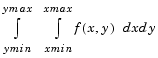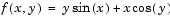MathematicsExample: Double Integration

Consider the numerical solution of

•For this example. The first step is to build the function to be evaluated. The function must be capable of returning a vector output when given a vector input. You must also consider which variable is in the inner integral, and which goes in the outer integral. In this example, the inner variable is x and the outer variable is y (the order in the integral is dxdy). In this case, the integrand function is

• ```function out = integrnd(x,y)
out = y*sin(x) + x*cos(y);
```

To perform the integration, two functions are available in the `funfun` directory. The first, `dblquad`, is called directly from the command line. This M-file evaluates the outer loop using `quad`. At each iteration, `quad` calls the second helper function that evaluates the inner loop.

To evaluate the double integral, use

• ```result = dblquad(@integrnd,xmin,xmax,ymin,ymax);
```

The first argument is a string with the name of the integrand function. The second to fifth arguments are

 `xmin` Lower limit of inner integral `xmax` Upper limit of the inner integral `ymin` Lower limit of outer integral `ymax` Upper limit of the outer integral

Here is a numeric example that illustrates the use of `dblquad`.

• ```xmin = pi;
xmax = 2*pi;
ymin = 0;
ymax = pi;
result = dblquad(@integrnd,xmin,xmax,ymin,ymax)
```

The result is -9.8698.

By default, `dblquad` calls `quad`. To integrate the previous example using `quadl` (with the default values for the tolerance argument), use

• ```result = dblquad(@integrnd,xmin,xmax,ymin,ymax,[],@quadl);
```

Alternatively, you can pass any user-defined quadrature function name to `dblquad` as long as the quadrature function has the same calling and return arguments as `quad`.Example: Computing the Length of a Curve Parameterizing Functions Called by Function Functions© 1994-2005 The MathWorks, Inc.